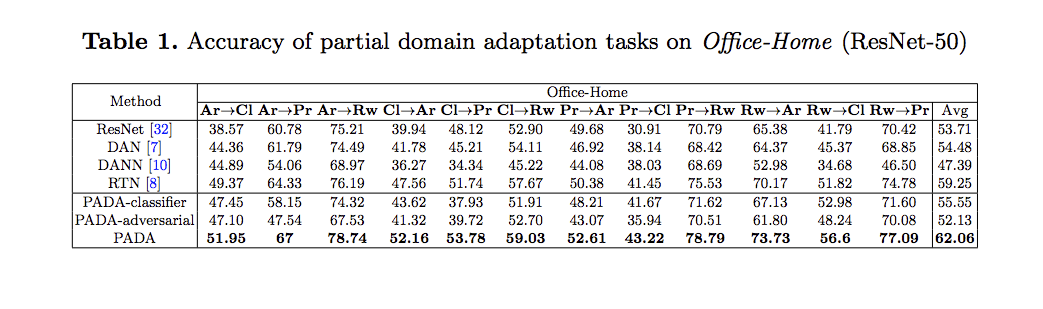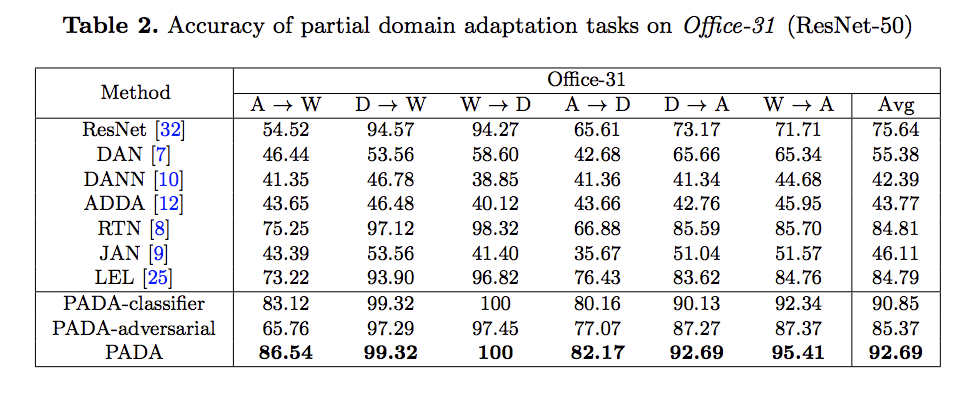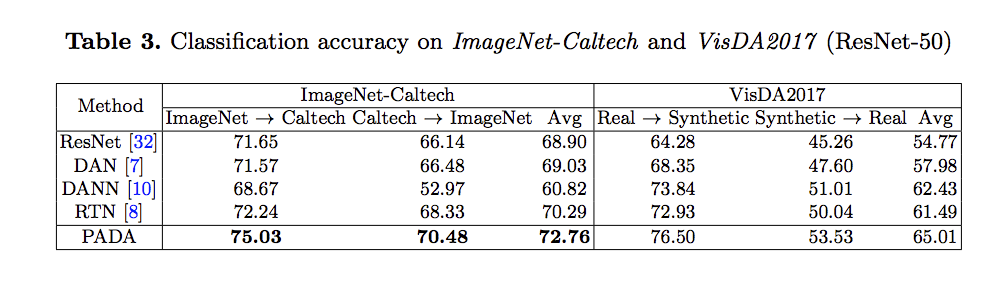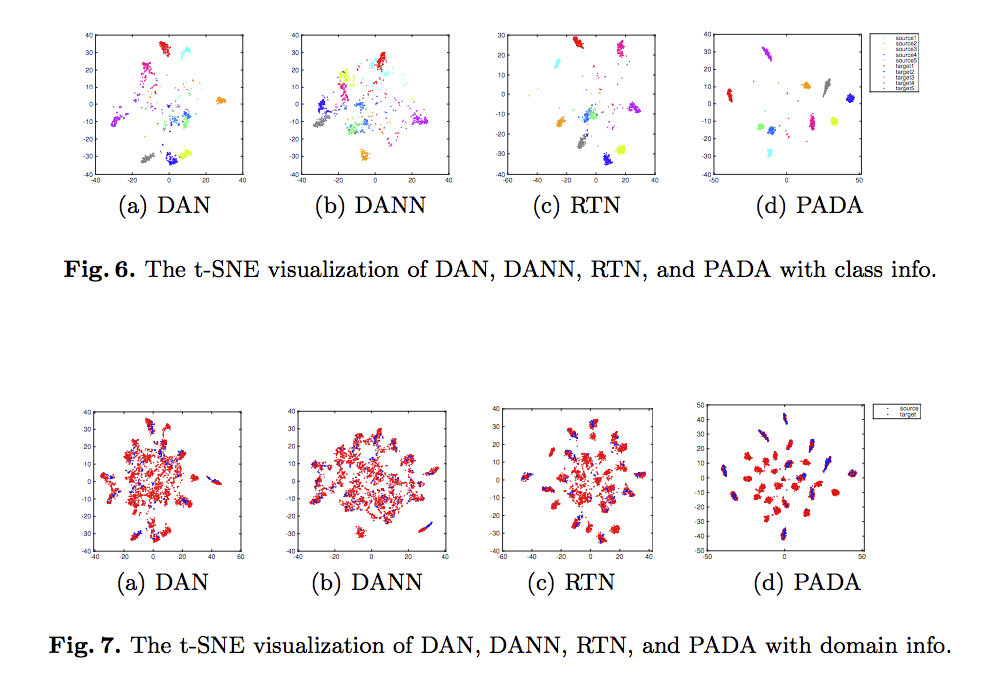Zhangjie Cao, Lijia Ma, Mingsheng Long, Jianmin Wang. “Partial Adversarial Domain Adaptation”. In ECCV’18.

ECCV 2018 paper.

# 簡介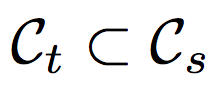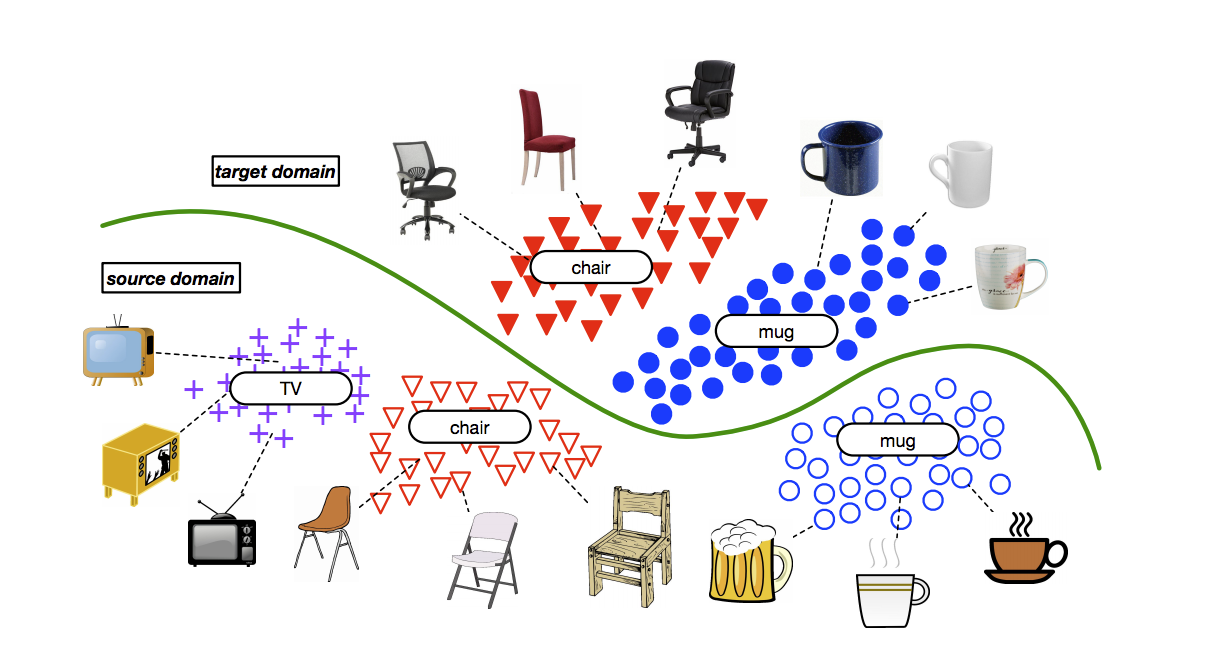Cs/Ct 指的是 Cs 的眾多類別中，不屬於 Ct 的類別，我們會寫作 Cs/Ct， 並且稱作 Outlier label space.

# 概念

 會讓模型偏好 Cs/Ct 的資料集而無法有效的對 Ct 進行遷移（（這邊給的前提是 Ct « Cs/Ct 。

• negative transfer - 訓練 Cs/Ct - outlier 的類別，可能會降低我們的準確度
• positive transfer - 針對 Ct 的類別，讓模型對於兩個資料集的分佈相近。

# 方法

• Gf: Feature Extractor
• Gy: Classifier
• Gd: Domain Discriminator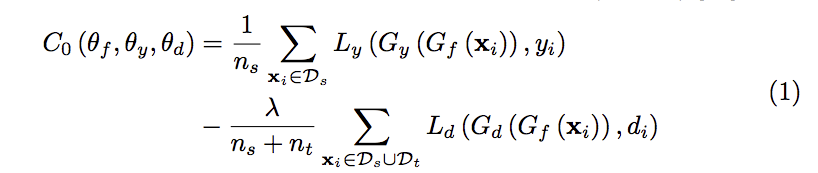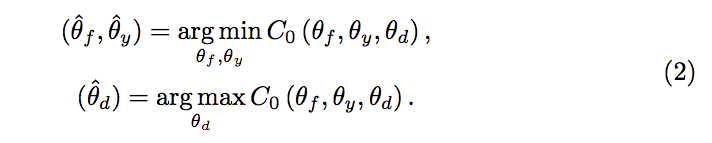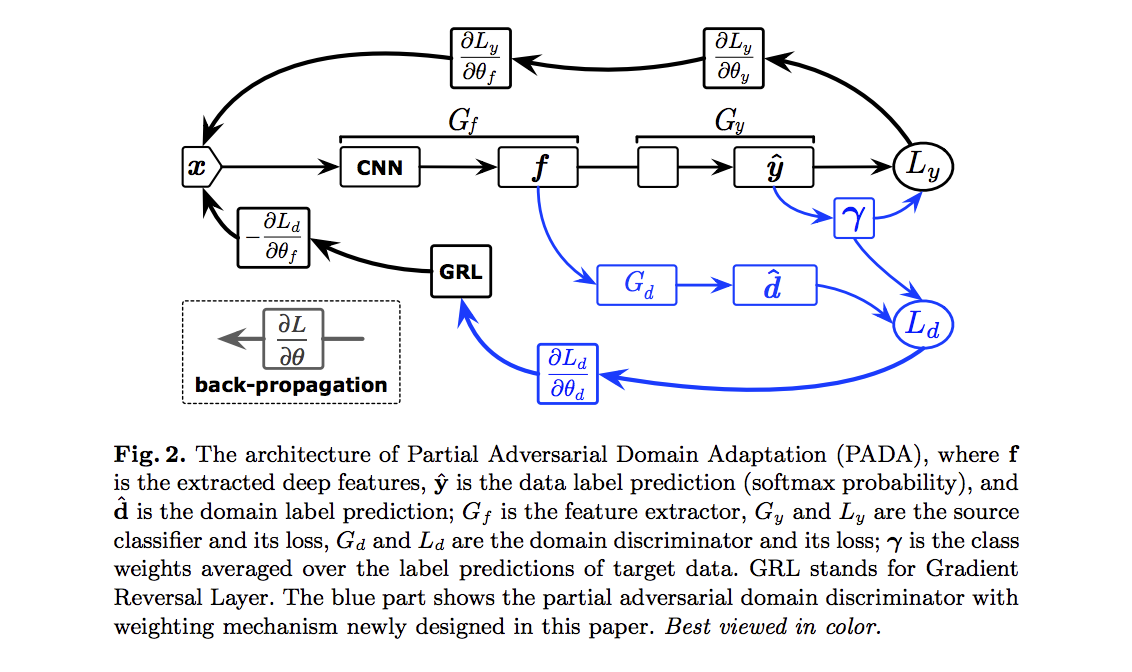Fortunately, we observe that the output of the source classifier yˆi = Gy(xi) to each data point xi gives a probability distribution over the source label space Cs.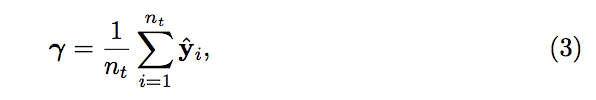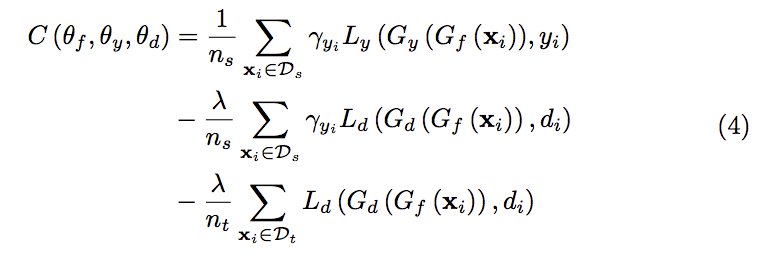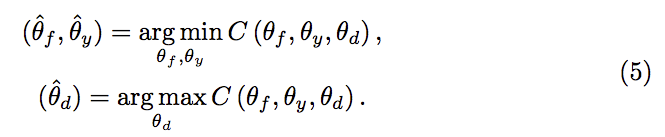# 程式碼部分

pyTorch 真方便。

class_weight = torch.mean(target_fc8_out, 0)
class_weight = (class_weight / torch.mean(class_weight)).cuda().view(-1)
class_criterion = nn.CrossEntropyLoss(weight = class_weight)



# 實驗